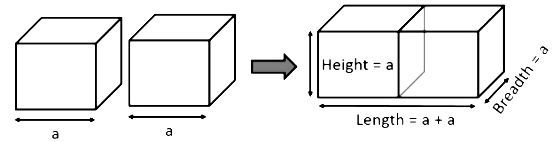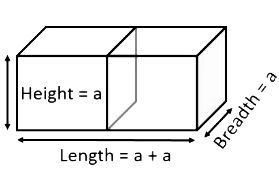Home/Class 10/Maths/

$$2$$ cubes each of volume $$64\;\;{cm}^{3}$$are joined end to end. Find the surface area of the resulting cuboid.Speed
00:00
03:48## QuestionMathsClass 10

$$2$$ cubes each of volume $$64\;\;{cm}^{3}$$are joined end to end. Find the surface area of the resulting cuboid.

The surface area of resulting cuboid is $$160\ cm^2$$
4.64.6## Solution

The diagram is given as:Given,
The Volume ($$V$$) of each cube is$$=64\;{cm}^{3}$$
$$\because Volume\;={\left. \left(side\right)\right. }^{3}$$
Let a be the side of the given cube , so,
$${a}^{3}=64$$
$$\Rightarrow a=4\;cm$$
Now the resulting cuboid will beThe length of cuboid (l)$$=a+a=4+4=8\;cm$$
breadth(b)$$=a=4\;cm$$
height(h)$$=a=4\;cm$$
So, Surface Area of Cuboid $$= 2(lb + bh + lh)$$ ;  where l is length ,b is breadth and h is height of the cuboid.
$$=2(8\times 4+4\times 4+4\times 8)\;{cm}^{2}$$
$$=2(32+16+32)\;{cm}^{2}$$
$$=(2\times 80)\;{cm}^{2}=160\;{cm}^{2}$$
Thus , surface area of cuboid is $$160\;{cm}^{2}$$.